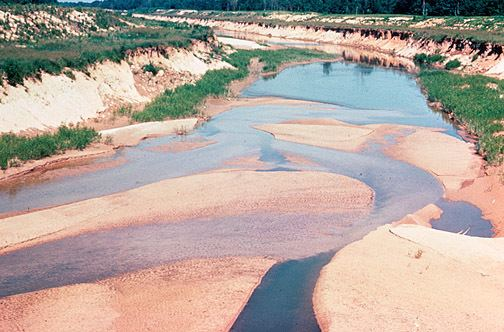# Sedimentation

Updated on
Covid-19Sedimentation is the tendency for particles in suspension to settle out of the fluid in which they are entrained and come to rest against a barrier. This is due to their motion through the fluid in response to the forces acting on them: these forces can be due to gravity, centrifugal acceleration, or electromagnetism. In geology, sedimentation is often used as the opposite of erosion, i.e., the terminal end of sediment transport. In that sense, it includes the termination of transport by saltation or true bedload transport. Settling is the falling of suspended particles through the liquid, whereas sedimentation is the termination of the settling process. In estuarine environments, settling can be influenced by the presence or absence of vegetation. Trees such as mangroves are crucial to the attenuation of waves or currents, promoting the settlement of suspended particles.

## Contents

Sedimentation may pertain to objects of various sizes, ranging from large rocks in flowing water to suspensions of dust and pollen particles to cellular suspensions to solutions of single molecules such as proteins and peptides. Even small molecules supply a sufficiently strong force to produce significant sedimentation.

The term is typically used in geology to describe the deposition of sediment which results in the formation of sedimentary rock, but it is also used in various chemical and environmental fields to describe the motion of often-smaller particles and molecules. This process is also used in the biotech industry to separate cells from the culture media.

## Experiments

In a sedimentation experiment, the applied force accelerates the particles to a terminal velocity v t e r m at which the applied force is exactly canceled by an opposing drag force. For small enough particles (low Reynolds number), the drag force varies linearly with the terminal velocity, i.e., F d r a g = f v t e r m (Stokes flow) where f depends only on the properties of the particle and the surrounding fluid. Similarly, the applied force generally varies linearly with some coupling constant (denoted here as q) that depends only on the properties of the particle, F a p p = q E a p p . Hence, it is generally possible to define a sedimentation coefficient s   = d e f   q / f that depends only on the properties of the particle and the surrounding fluid. Thus, measuring s can reveal underlying properties of the particle.

In many cases, the motion of the particles is blocked by a hard boundary; the resulting accumulation of particles at the boundary is called a sediment. The concentration of particles at the boundary is opposed by the diffusion of the particles.

The sedimentation of a single particle under gravity is described by the Mason–Weaver equation, which has a simple exact solution. The sedimentation coefficient s in this case equals m b / f , where m b is the buoyant mass.

The sedimentation of a single particle under centrifugal force is described by the Lamm equation, which likewise has an exact solution. The sedimentation coefficient s also equals m b / f , where m b is the buoyant mass. However, the Lamm equation differs from the Mason–Weaver equation because the centrifugal force depends on radius from the origin of rotation, whereas in the Mason–Weaver equation gravity is constant. The Lamm equation also has extra terms, since it pertains to sector-shaped cells, whereas the Mason–Weaver equation is one-dimensional.

Classification of sedimentation:

• Type 1 sedimentation is characterized by particles that settle discretely at a constant settling velocity,or by the deposition of Iron-Rich minerals to streamlines down to the point source. They settle as individual particles and do not flocculate or stick to other during settling. Example: sand and grit material
• Type 2 sedimentation is characterized by particles that flocculate during sedimentation and because of this their size is constantly changing and therefore their settling velocity is changing. Example: alum or iron coagulation
• Type 3 sedimentation is also known as zone sedimentation. In this process the particles are at a high concentration (greater than 1000 mg/L) such that the particles tend to settle as a mass and a distinct clear zone and sludge zone are present. Zone settling occurs in lime-softening, sedimentation, active sludge sedimentation and sludge thickeners.
• ## Geology

In geology, sedimentation is the deposition of particles carried by a fluid flow. For suspended load, this can be expressed mathematically by the Exner equation, and results in the formation of depositional landforms and the rocks that constitute sedimentary record. An undesired increased transport and sedimentation of suspended material is called siltation, and it is a major source of pollution in waterways in some parts of the world. High sedimentation rates can be a result of poor land management and a high frequency of flooding events. If not managed properly, it can be detrimental to fragile ecosystems on the receiving end, such as coral reefs. Climate change also affects siltation rates.

## Chemistry

In chemistry, sedimentation has been used to measure the size of large molecules (macromolecule), where the force of gravity is augmented with centrifugal force in an ultracentrifuge.

Topics